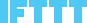## Thursday, April 14, 2016

### Division the long way

My colleague, Bob Doran, brought this to my attention and he writes: Recently, a video appeared on Youtube purporting to show a Facit electro-mechanical calculator deriving pi.  In fact, the calculator is merely dividing 126358 by 40221. This gives a good approximation to pi. 126358/40221 = 3.14159270, whereas pi is 3.14159265. However, the video does give a neat demonstration of an algorithm called "non-restoring division".

Calculators are really just simple adders that add two numbers in one revolution of the mechanism. These adders can also subtract if rotated the other way. Calculators may perform other simple actions such shifting numbers to the left or right, entering data, clearing registers and counting up or down. But to perform division (or multiplication) any simple calculator has to follow a program of many steps – a mechanical program, but a program none-the-less.
Consider how the few of us who can still do division with pencil and paper go about it. We would look at the dividend 126358 and the divisor 40221 and use our memorized "gzinta" tables to guess the first digit of the quotient i.e. "4 goes into 12" 3 times. We then form 3 x 40221 = 120663 and, as that is less than 126358, subtract it, then "take a step to the right" and continue. Most modern computers divide in much the same way, having a built-in "quotient digit predictor". However, older computers, didn't have such intelligence – they needed to subtract to even compare two numbers, unlike ourselves who can just see with one glance that 120663 is less that 126358. Older computers and calculators had to work out the next quotient digit by subtracting the divisor again and again from the dividend, keeping count, until the result of a subtraction became negative, showing it had gone too far and thus needing to restore the dividend by adding the divisor once (and reducing the count by 1 to give the correct quotient digit.)
This is where non-restoring division comes in. When the result of a subtraction becomes negative it means that we have subtracted the divisor an extra one time at this quotient digit place. This is the same as having subtracted an extra ten times one place to the right. So, rather than adding to restore immediately, the program says to move to the right and then continue by adding the divisor (and counting down) rather than subtracting. When the dividend becomes positive we have found the next quotient digit, so can move to the right again and subtract the divisor repeatedly – and so on.
Look at the video again and now notice that the direction of rotation changes for each quotient digit produced. Clever, eh?

from The Universal Machine http://universal-machine.blogspot.com/Put the internet to work for you.